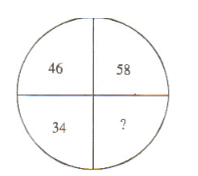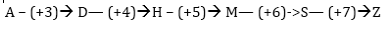# Railway JE General intelligence & Reasoning quiz for (Phase I) and other railways exams 11/02/2019

## Railway JE General intelligence & Reasoning quiz for (Phase I)

General intelligence & Reasoning quiz for RRB JE(phase I) and other railways exam, and other competitive exams.

Q1. In the following questions, select the related letters/ word/ number from the given alternatives.
Taste : Tongue : : Walk : ?
A) Pavement
B) Crutch
C) Legs
D) Walking sticky
Q2. In the following questions, select the related letters/ word/ number from the given alternatives.
Chimney : Smoke : : _____ : ____
A) Gun : Bullet
B) House : Roof
C) Clay : Ceramic
D) Tea : Kettle

Q3. In the following questions, select the related letters/ word/ number from the given alternatives.
DCHG : LKPO : : FEJI : ?
A) NMRU
B) NMRQ
C) MNRQ
D) OPQR
Q4. In the following questions, select the related letters/ word/ number from the given alternatives.
5 : 124 : : 7 : ?
A) 342
B) 343
C) 248
D) 125
Q5. In each of the following questions, select the one which is different from the other three responses.
A) Eyes
B) Hand
C) Nose
D) Ear
Q6. In the following questions, a series is given with one term missing. Choose the correct alternative from the given ones that will complete the series.
2, 5, 10, 17, 26, 37, 50, ?
A) 63
B) 65
C) 67
D) 69
Q7. In the following questions, a series is given with one term missing. Choose the correct alternative from the given ones that will complete the series.
A, D, H, M, ? , Z
A) B
B) G
C) S
D) N
Q8. Which one set of the letter when sequentially placed at the gaps in the given letter series shall complete it?
_op_mo_n_ _ pnmop_
A) mnompn
B) mnpomn
C) mpnmop
D) mnpmon
Q9. In the following question, arrange the given words in a meaningful sequence and then choose the most appropriate sequence from among the alternatives provided below each question:
a. Birth
b. Death
c. Childhood
d. Infancy
g. Old age
A) b, f, g, e, d, c, a
B) a, d, c, e, f, g, b
C) a, d, c, f, e, g, b
D) b, g, f, d, e, c, a
Q10. Select the missing number from the given responses.A) 92
B) 72
C) 22
D) 68

# Solutions

Q1. Ans(C)
Tongue is used to taste something. Similarly, leg is used to walk.

Q2. Ans(A)
Smoke is emitted through chimney. Similarly, bullet is fired from gun.

Q3. Ans(B)
+8,+8,+8,+8 SERISE
So, ? = NMRQ

Q4. Ans(A)
(5)³ – 1 = 124
Similarly, (7)³ – 1 = 342

Q5. Ans (B)
Except Hand, all others are sense organs.

Q6. Ans(B)
The series are +3 , +5 , + 7, + 9 , + 11 , + 13 , + 15
So, ? = 50 + 15 = 65

Q7. Ans(D)Q8. Ans(D)
mopn/mopn/mopn/mopn

Q9. Ans(B)Q10. Ans(C)
Subtract 12 from the preceding number and proceed anticlockwise.
58 – 12 = 46;
46 – 12 = 34
34 – 12 = 22
So, ? = 22

## WhatsApp Group Join here

Mail us at : ambitiousbaba1@gmail.com